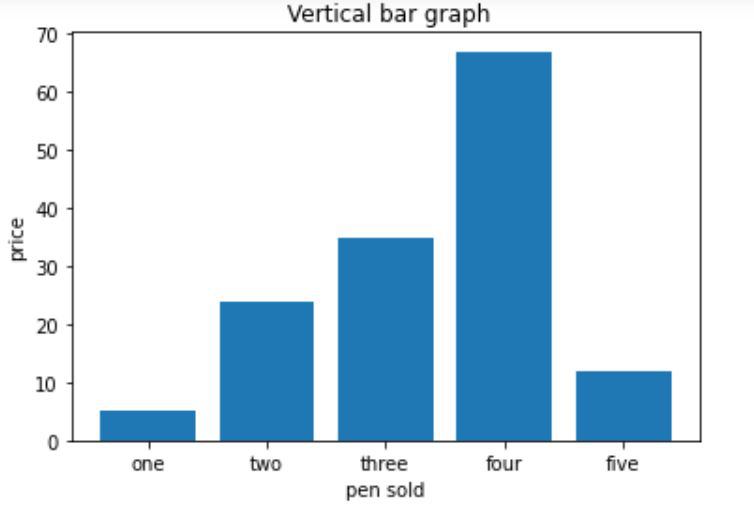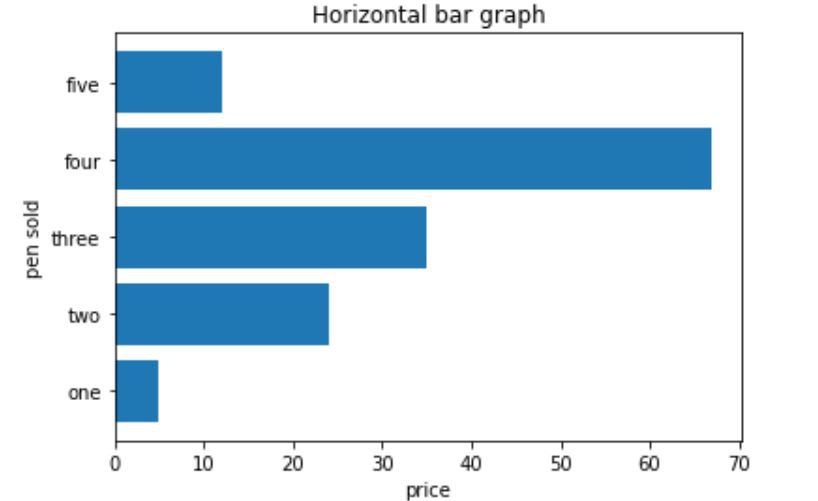Open in App
Not now

## Related Articles

• Write an Interview Experience

# Draw a horizontal bar chart with Matplotlib

• Difficulty Level : Medium
• Last Updated : 25 Aug, 2021

Matplotlib is the standard python library for creating visualizations in Python. Pyplot is a module of Matplotlib library which is used to plot graphs and charts and also make changes in them. In this article, we are going to see how to draw a horizontal bar chart with Matplotlib.

### Creating a vertical bar chart

Approach:

• Importing matplotlib.pyplot as plt
• Creating list x for discrete values on x-axis
• Creating list y  consisting only numeric data for discrete values on y-axis
• Calling plt.bar() function with parameters x,y as plt.bar(x,y)
• Setting x_label() and y_label()
• Setting title() for our bar chart
• Calling plt.show() for visualizing our chart

Below is the implementation:

## Python3

 `import` `matplotlib.pyplot as plt` `x``=``[``'one'``, ``'two'``, ``'three'``, ``'four'``, ``'five'``]` `# giving the values against``# each value at x axis``y``=``[``5``, ``24``, ``35``, ``67``, ``12``]``plt.bar(x, y)` `# setting x-label as pen sold``plt.xlabel(``"pen sold"``)` `# setting y_label as price``plt.ylabel(``"price"``)  ``plt.title(``" Vertical bar graph"``)``plt.show()`

Output:### Creating a horizontal bar chart

Approach:

• Importing matplotlib.pyplot as plt
• Creating list y   for discrete values on y-axis
• Creating list x  consisting only numeric data for discrete values on x-axis
• Calling plt.barh() function with parameters y,x as plt.barh(y,x)
• Setting x_label() and y_label()
• Setting title() for our bar chart
• Calling plt.show() for visualizing our chart

Below is the implementation:

## Python3

 `import` `matplotlib.pyplot as plt``y``=``[``'one'``, ``'two'``, ``'three'``, ``'four'``, ``'five'``]` `# getting values against each value of y``x``=``[``5``,``24``,``35``,``67``,``12``]``plt.barh(y, x)` `# setting label of y-axis``plt.ylabel(``"pen sold"``)` `# setting label of x-axis``plt.xlabel(``"price"``)``plt.title(``"Horizontal bar graph"``)``plt.show()`

Output:My Personal Notes arrow_drop_up